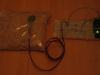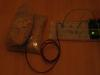# Theory and Practice of Tangible User Interfaces

### Announcements

November 24, 2007
Reading for November 27th, are now posted. Enjoy!

October 2, 2007
To upload your thoughtless acts, create a new assignment page like any other lab. You'll see "Thoughtless Acts" listed as one of the assignment options.

May 24, 2008
This site has been archived and is no longer editable. Stay tuned for the next version, coming in the fall!

# FSR Visualization and Mechanical Construction

Project Members:
Jess Kline

### Part 1: Programming

#### Description

I used the modified arduino ball code and added more ellipses to the visualization (10 to be exact). Initially, I tried to replace the original ellipse with a clock visualization found on the Processing website but could not incorporate this code into the existing code.

#### Components

Lab 4 setup: breadboard, arduino, green LED, resistors, yellow green and red wires, force sensitive resistor

#### Code

import processing.serial.*;
String portname = "COM4";
Serial port;
String buf="";
int cr = 13;
int lf = 10;
void setup() {
size(300,300);
frameRate(10);
smooth();
background(40,40,40);
noStroke();
port = new Serial(this, portname, 9600);
}
void draw() {
}
void drawball(int a, int b, int d, int e, int f, int g, int h,
int j, int k, int l, int m, int n, int o, int q,
int s, int t, int u, int v, int w, int x, int y, int z, int r) {
for (int i=0; i<100; i++ ) {
fill(255-i,i,240);
ellipse(a,b,r,r);
ellipse(d,e,r,r);
ellipse(f,g,r,r);
ellipse(h,j,r,r);
ellipse(k,l,r,r);
ellipse(m,n,r,r);
ellipse(o,q,r,r);
ellipse(s,t,r,r);
ellipse(u,v,r,r);
ellipse(w,x,r,r);
ellipse(y,z,r,r);
}
}
void serialEvent(Serial p) {
if (c != lf && c != cr) {
buf += char(c);
}
if (c == lf) {
int val = int(buf);
println("val="+val);
int a = int(random(0,width));
int b = int(random(0,height));
int d = int(random(0,width));
int e = int(random(0,height));
int f = int(random(0,width));
int g = int(random(0,height));
int h = int(random(0,width));
int j = int(random(0,height));
int k = int(random(0,width));
int l = int(random(0,height));
int m = int(random(0,width));
int n = int(random(0,height));
int o = int(random(0,width));
int q = int(random(0,height));
int s = int(random(0,width));
int t = int(random(0,height));
int u = int(random(0,width));
int v = int(random(0,height));
int w = int(random(0,width));
int x = int(random(0,height));
int y = int(random(0,width));
int z = int(random(0,height));
drawball(a,b,d,e,f,g,h,j,k,l,m,n,o,q,s,t,u,v,w,x,y,z,val);
buf = "";
background(40,40,40);
}
}

### Part 2: Mechanical

#### Description

I made a force distributor by pouring rice into a ziplock bag and wrapping the bag around the FSR. This distributor distributes the physical force over a greater area. The bag of rice is also a more comfortable surface to exert force because you can grip and squeeze the distributor in your hand rather than positioning the small sensor between two fingers.

#### Components

• Lab 4 setup: breadboard, arduino, green LED, resistors, yellow green and red wires
• Force sensitive resistor with soldered wires
• Force distributor: basmati and jasmine rice, sandwich-sized ziplock bag, scotch tape

#### Code

/*
* Resistive Sensor Input
* Takes the input from a resistive sensor, e.g., FSR or photocell
* Dims the LED accordingly, and sends the value (0-255) to the serial port
*/
int sensorPin = 0; // select the input pin for the sensor
int ledPin = 11; // select the output pin for the LED
int val = 0; // variable to store the value coming from the sensor
void setup() {
Serial.begin(9600);
}
void loop() {
analogWrite(ledPin, val/4); // analogWrite (dimming the LED) can be between 0-255
Serial.println(val/4); // writing the value to the PC via serial connection
delay(50); // rest a little...
}

#### PicturesDistributor setup part 1Distributor setup part 2